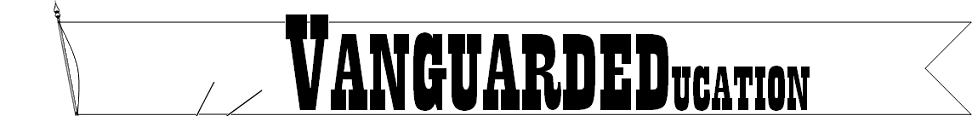•• Home
•• Curriculum
•• Shopping
•• Free Items
••• Contact
•There are six maths practice pages available for download. Three of the math practice pages are A4 paper size and the other three are 8.5X11 paper size. If you are in North America, select the 8.5X11 paper size. The rest of the world uses the A4 paper size.
The three simple math practice pages are addition, subtraction, and combined addition and subtraction. Each free math download consists of seven pages of practice for the student.
The addition problems have a sum which is ten or less. The subtraction problems use numbers 0-10. The combined addition and subtraction maths practice are a combination of the addition and subtraction problems just described.
Select the image above for the download you would like, or click on the links below. When you select one of the downloads, you will be given the option of opening the file or saving it. If you open it, you can print directly from the web page. If you download the simple maths practice pages, the PDF file can be found in the downloads folder of your computer.

Subtraction practice 8.5X11

Combined Addition and Subtraction practice 8.5X11